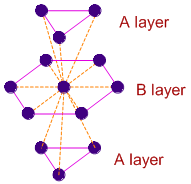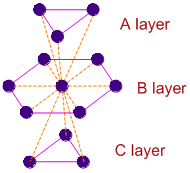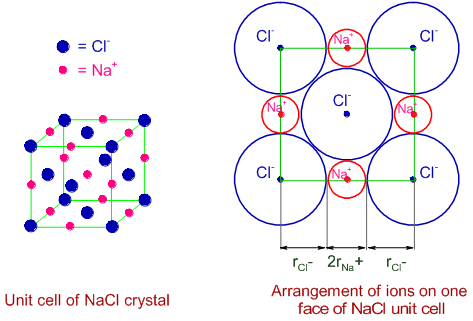< Previous question Next question >

# COORDINATION NUMBER IN CRYSTALS IIT JEE MCQ

## 1) The coordination number of a metal crystallizing in a hexagonal close-packed structure is:

(IIT JEE 2000)

a) 12

b) 4

c) 8

d) 6

Logic and Solution:

In three dimensional hexagonal close packing of crystals, the two dimensional hcp layers are arranged in ABAB........ pattern as shown below. The atoms in A layers are exactly above (or below) one type of the trigonal voids in B layer.

The central atom in B layer is immediately surrounded by 12 atoms i.e., 6 in layer B and 3 each in two A layers.Conclusion:

The correct option is "a".

Extra information:

In Cubic Close Packing (ccp or fcc) also the coordination number if 12. In this case the two dimensional hcp layers are arranged in ABCABC....... pattern as shown below. The atoms in layer C are arranged below second type of trigonal voids of layer B. However, the number of nearest atoms around the central atom in B layer is same i.e., 12.## Related questions

### 2) Which of the following statements is (are) correct?

(IIT JEE 1998)

1) The coordination number of each type of ion in CsCl crystal is 8.

2) A metal that crystallizes in BCC structure has coordination number equal to 12.

3) A unit cell of an ionic crystal shares some of its ions with other unit cells.

4) The length of unit cell in NaCl is 552 pm. [ rNa+ = 95 pm and rCl-=181 pm]

Solution:

Option-1:

CsCl crystal structure along with coordination is show below. The Cs+ ion is surrounded by 8 Cl- ions at the corners of unit cell. It is also clearly shown in the extended unit cells that the Cl- ion is also surrounded by 8 Cs+ ions. Therefore option '1' is correct.Option-2:

BCC structure of metals is shown below. The metal atom at the center of the body is surrounded by 8 metal atoms that are at the corners of the cube. Hence option '2' is incorrect.Option-3:

Every unit cell shares some of its atoms/ions with other unit cells. So option '3' is correct.

Option-4:

Unit cell of NaCl is shown below. The Cl- ions are arranged at the corners as well as at the centers of the faces, while the Na+ ions occupy the centers of the edges along with a position at the body center.The Na+ and Cl- ions are touching each other along the edge of the unit cell.

Hence the edge length  = (2rNa+) + (2rCl-) = (2 x 95) + (2 x 181) = 552 pm.

Therefore option '4' is also correct.

Conclusion:

The correct answer is: option "3".

## Homework

1)

 < Previous question Next question >

Author: Aditya vardhan Vutturi